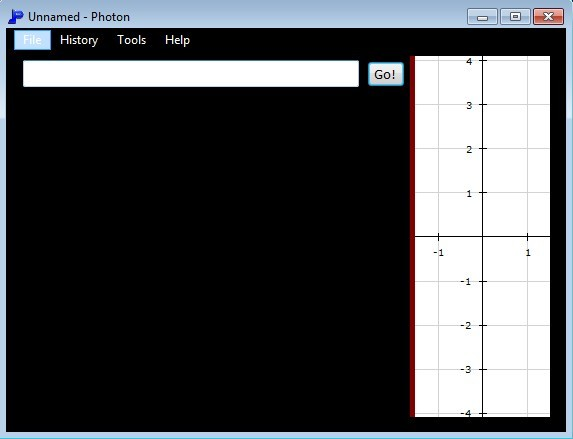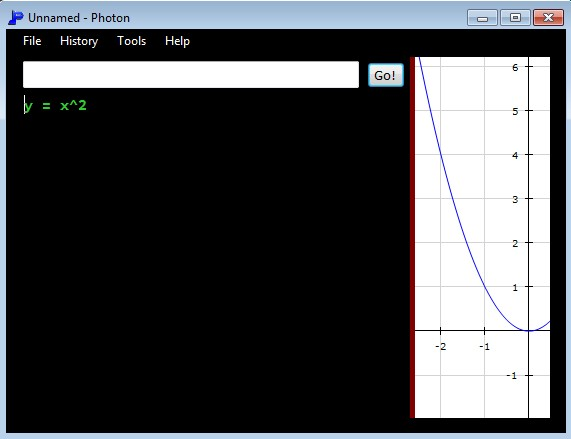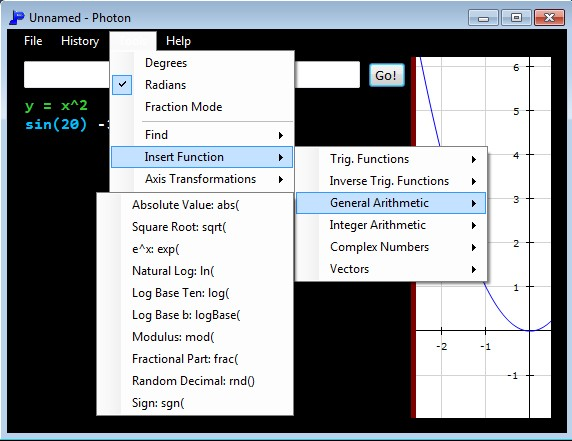Editor Ratings:
User Ratings:
[Total: 0 Average: 0]

Photon is a free graphing calculator software for Windows which you can use to plot mathematical functions. It comes with a selection of built-in functions (trigonometric, general arithmetic, complex numbers, vectors, integer arithmetic and so on) that you can plot out as examples or alternatively, functions can be typed into the calculator manually and then plotted out.

Input field at the top is where you write mathematical functions, the ones that you would like to see plotted. Plotting area can be seen on the right. It might seem small, and it is, but you can navigate through it by left clicking, holding down the left click and then moving your mouse around to whichever point of the function you’d like to examine. Built-in functions that you can test out are accessible from the top left menu.Similar software: Functy.

## Key features of Photo free graphing calculator are:

• Portable – doesn’t require installation, just click in order to run
• Comes with built-in example mathematical functions that you can use
• Allows you to calculate trigonometric functions, vectors, complex#, etc.
• Supports three different modes of work degrees, radians and fractions
• Has a quick find tool for zero, minimum, maximum and inflection point
• Export plotted functions as PNG, GIF, BMP, JPEG, TXT or Photon file

Last of the supported formats, Photon, is Photos’s own format which is editable. This means that you can save created graphs and then continue editing them later on. Graphing calculators are a must for high school and college students. If some of you would like to plot mathematical functions on your computer, you can do it with Photon. Here’s a few tips to help you get started.

## How to plot mathematical functions with Photon free graphing calculator for Windows

The only thing that you need to do in order to start plotting your function is to type it in the input field at the top and then click on the “Go” button.We typed in a very simple function, y = x^2, and right away after we clicked Go, it was plotted in the graphing area on the right. Only a small section of the graph can be seen, but like we said, you can easily navigate through it or use the find tool to find the points that you’re interested in. To insert example functions, click Help >> Examples.Additional calculations which are supported can be seen on the image above. They can be accessed by clicking on Tools >> Insert Functions. This is where you can find various other function tweaks, or you can change the mode of work from the three that are supported, this is also where you can access Options.

## Conclusion

Despite its slightly simplistic interface Photon has a lot of different tools and options for plotting mathematical functions. We only used a simplistic function in our example, but a lot more complicated ones can also be plotted and calculated. It’s portable, so if you’re only interested in testing it out, you don’t have to install anything.